Bicupola (geometry)

﻿
Bicupola (geometry)
Set of bicupolae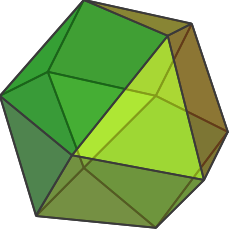Faces 2n triangles,
2n squares
2 n-agon
Edges 8n
Vertices 4n
Symmetry group Ortho: Dnh
Gyro: Dnd
Properties convex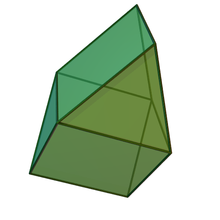The gyrobifastigium (J26) can be considered a digonal gyrobicupola.

In geometry, a bicupola is a solid formed by connecting two cupolae on their bases.

There are two classes of bicupola because each cupola half is bordered by alternating triangles and squares. If similar faces are attached together the result is an orthobicupola; if squares are attached to triangles it is a gyrobicupola.

Cupolae and bicupolae categorically exist as infinite sets of polyhedra, just like the pyramids, bipyramids, prisms, and trapezohedra.

Six bicupolae have regular polygon faces: triangular, square and pentagonal ortho- and gyrobicupolae. The triangular gyrobicupola is an Archimedean solid, the cuboctahedron; the other five are Johnson solids.

Bicupolae of higher order can be constructed if the flank faces are allowed to stretch into rectangles and isosceles triangles.

Bicupolae are special in having four faces on every vertex. This means that their dual polyhedra will have all quadrilateral faces. The best known example is the rhombic dodecahedron composed of 12 rhombic faces. The dual of the ortho-form, triangular orthobicupola, is also a dodecahedron, similar to rhombic dodecahedron, but it has 6 trapezoid faces which alternate long and short edges around the circumference.

Forms

Set of orthobicupolae

Symmetry Picture Description
D3h
[2,3]
*223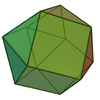Triangular orthobicupola (J27): 8 triangles, 6 squares; its dual is the trapezo-rhombic dodecahedron
D4h
[2,4]
*224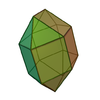Square orthobicupola (J28): 8 triangles, 10 squares
D5h
[2,5]
*225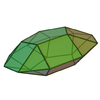Pentagonal orthobicupola (J30): 10 triangles, 10 squares, 2 pentagons
Dnh
[2,n]
*22n
n-gonal orthobicupola: 2n triangles, 2n squares, 2 n-gons

Set of gyrobicupolae

Symmetry Picture Description
D2d
[2+,4]
2*2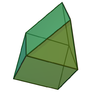Gyrobifastigium (J26): 4 triangles, 4 squares
D3d
[2+,6]
2*3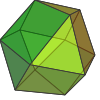Triangular gyrobicupola or cuboctahedron: 8 triangles, 6 squares; its dual is the rhombic dodecahedron
D4d
[2+,8]
2*4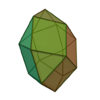Square gyrobicupola (J29): 8 triangles, 10 squares
D5d
[2+,10]
2*5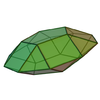Pentagonal gyrobicupola (J31): 10 triangles, 10 squares, 2 pentagons
Dnd
[2+,2n]
2*n
n-gonal gyrobicupola: 2n triangles, 2n squares, 2 n-gons

Wikimedia Foundation. 2010.

Look at other dictionaries:

• Gyroelongated square bicupola — Infobox Polyhedron Polyhedron Type=Johnson J44 J45 J46 Face List=3.8 triangles 2+8 squares Edge Count=56 Vertex Count=24 Symmetry Group= D 4 Vertex List=8(3.43) 2.8(34.4) Dual= Property List=convex, chiralIn geometry, the gyroelongated square… …   Wikipedia

• Gyroelongated pentagonal bicupola — Infobox Polyhedron Polyhedron Type=Johnson J45 J46 J47 Face List=3.10 triangles 10 squares 2 pentagons Edge Count=70 Vertex Count=30 Symmetry Group= D 5 Vertex List=10(3.4.5.4) 2.10(34.4) Dual= Property List=convex, chiralIn geometry, the… …   Wikipedia

• Gyroelongated triangular bicupola — Infobox Polyhedron Polyhedron Type=Johnson J43 J44 J45 Face List=2+3.6 triangles 6 squares Edge Count=42 Vertex Count=18 Symmetry Group=D3 Vertex List=6(3.4.3.4) 2.6(34.4) Dual= Property List=convex, chiralIn geometry, the gyroelongated… …   Wikipedia

• Prism (geometry) — For other uses, see Prism (disambiguation). Set of uniform prisms (A hexagonal prism is shown) Type uniform polyhedron Faces 2+n total: 2 {n} n {4} Edges …   Wikipedia

• Cupola (geometry) — For other uses, see cupola (disambiguation). Pentagonal cupola (example) Type Set of cupolas Faces n triangles, n squares 1 n agon, 1 2n agon Edges …   Wikipedia

• List of mathematics articles (B) — NOTOC B B spline B* algebra B* search algorithm B,C,K,W system BA model Ba space Babuška Lax Milgram theorem Baby Monster group Baby step giant step Babylonian mathematics Babylonian numerals Bach tensor Bach s algorithm Bachmann–Howard ordinal… …   Wikipedia

• List of mathematics articles (G) — NOTOC G G₂ G delta space G networks Gδ set G structure G test G127 G2 manifold G2 structure Gabor atom Gabor filter Gabor transform Gabor Wigner transform Gabow s algorithm Gabriel graph Gabriel s Horn Gain graph Gain group Galerkin method… …   Wikipedia

• Johnson solid — The elongated square gyrobicupola (J37), a Johnson solid …   Wikipedia

• Polyhedron — Polyhedra redirects here. For the relational database system, see Polyhedra DBMS. For the game magazine, see Polyhedron (magazine). For the scientific journal, see Polyhedron (journal). Some Polyhedra Dodecahedron (Regular polyhedron) …   Wikipedia

• Gyroelongated square cupola — Infobox Polyhedron Polyhedron Type=Johnson J 22 J23 J24 Face List=3.4+8 triangles 1+4 squares 1 octagon Edge Count=44 Vertex Count=20 Symmetry Group= C 4v| Vertex List=4(3.43) 2.4(33.8) 8(34.4) Dual= Property List=convexIn geometry, the… …   Wikipedia